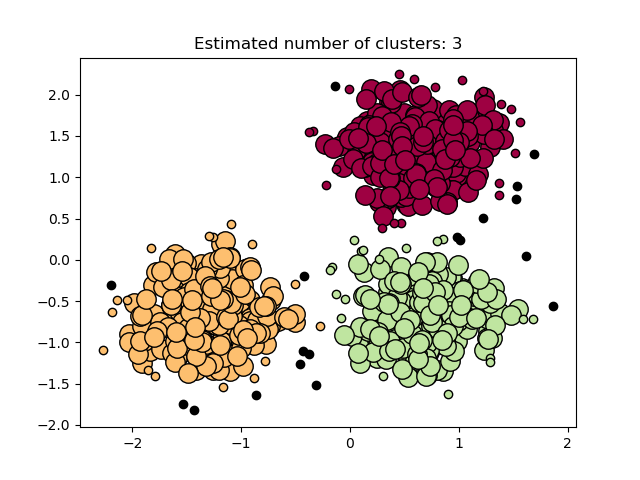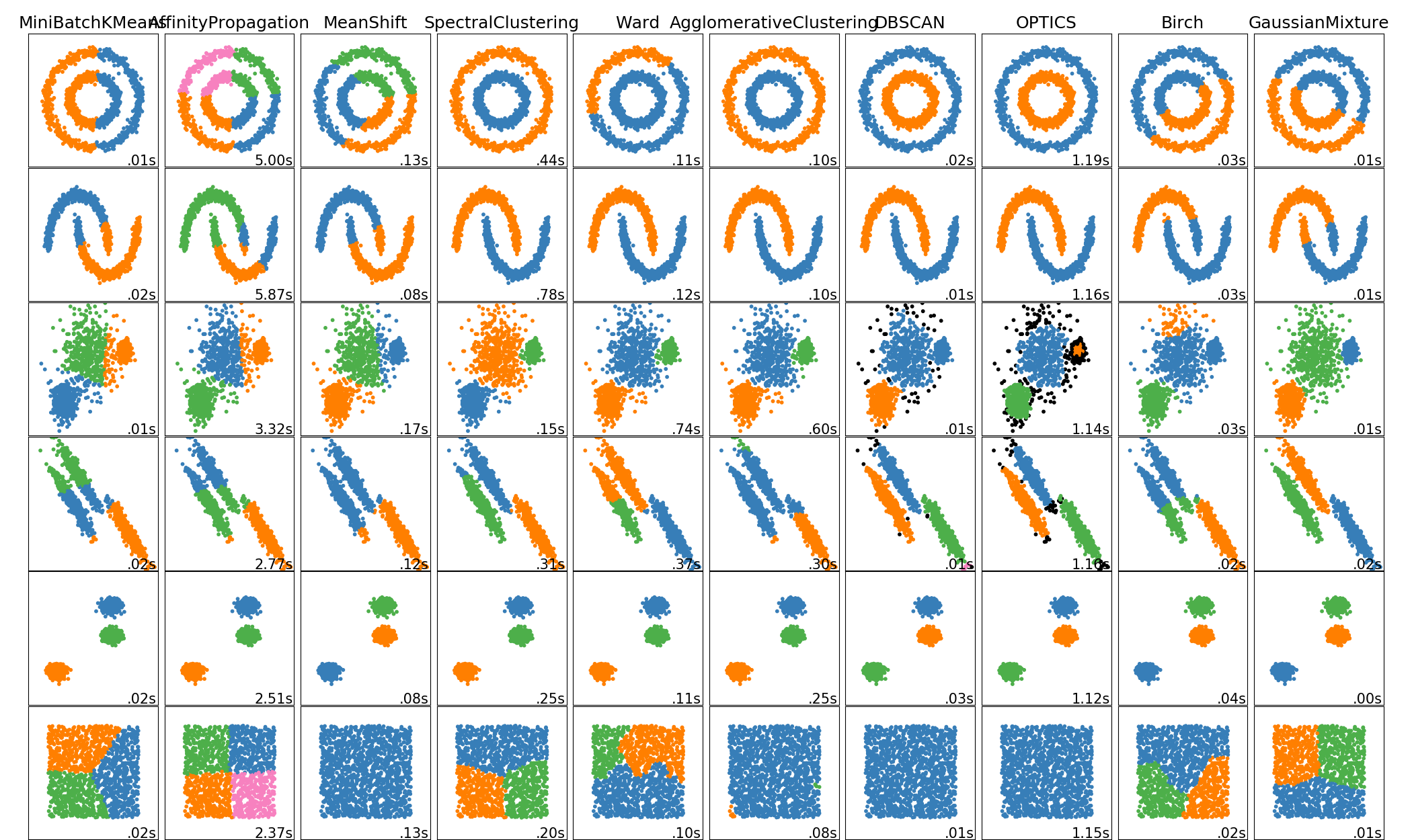# sklearn.cluster.DBSCAN¶

class sklearn.cluster.DBSCAN(eps=0.5, *, min_samples=5, metric='euclidean', metric_params=None, algorithm='auto', leaf_size=30, p=None, n_jobs=None)

[源码]

DBSCAN-基于密度的带噪声应用空间聚类。发现高密度的核心样本并从中膨胀团簇。对于包含类似密度的簇的数据来说是很好的。

eps float, default=0.5

min_samples int, default=5

metric string, or callable, default=’euclidean’

metric_params dict, default=None

algorithm {‘auto’, ‘ball_tree’, ‘kd_tree’, ‘brute’}, default=’auto’
NearestNeighbors模块用于计算点态距离和寻找最近邻的算法。有关详细信息，请参阅NearestNeighbors模块文档。
p float, default=None

n_jobs int, default=None

core_sample_indices_ ndarray of shape (n_core_samples,)

components_ ndarray of shape (n_core_samples, n_features)

labels_ ndarray of shape (n_samples)

OPTICS

Ester, M., H. P. Kriegel, J. Sander, and X. Xu, “A Density-Based Algorithm for Discovering Clusters in Large Spatial Databases with Noise”. In: Proceedings of the 2nd International Conference on Knowledge Discovery and Data Mining, Portland, OR, AAAI Press, pp. 226-231. 1996

Schubert, E., Sander, J., Ester, M., Kriegel, H. P., & Xu, X. (2017). DBSCAN revisited, revisited: why and how you should (still) use DBSCAN. ACM Transactions on Database Systems (TODS), 42(3), 19.

>>> from sklearn.cluster import DBSCAN>>> import numpy as np>>> X = np.array([[1, 2], [2, 2], [2, 3],...               [8, 7], [8, 8], [25, 80]])>>> clustering = DBSCAN(eps=3, min_samples=2).fit(X)>>> clustering.labels_array([ 0,  0,  0,  1,  1, -1])>>> clusteringDBSCAN(eps=3, min_samples=2)

fit(self, X[, y, sample_weight]) 从特征或距离矩阵执行DBSCAN聚类
fit_predict(self, X[, y, sample_weight]) 从特征或距离矩阵执行DBSCAN聚类，并返回聚类标签。
get_params(self[, deep]) 获取此估计器的参数
set_params(self, **params) 设置此估计器的参数
__init__(self, eps=0.5, *, min_samples=5, metric='euclidean', metric_params=None, algorithm='auto', leaf_size=30, p=None, n_jobs=None)

[源码]

fit(self, X, y=None, sample_weight=None)

[源码]

X {array-like, sparse matrix} of shape (n_samples, n_features), or (n_samples, n_samples)

sample_weight array-like of shape (n_samples,), default=None

y Ignored

self -
fit_predict(self, X, y=None, sample_weight=None)

[源码]

X {array-like, sparse matrix} of shape (n_samples, n_features), or (n_samples, n_samples)

sample_weight array-like of shape (n_samples,), default=None

y Ignored

labels ndarray, shape (n_samples,)

get_params(self, deep=True)

[源码]

deep bool, default=True

params mapping of string to any

set_params(self, **params)

[源码]

**params dict

self object

## sklearn.cluster.DBSCAN使用示例¶